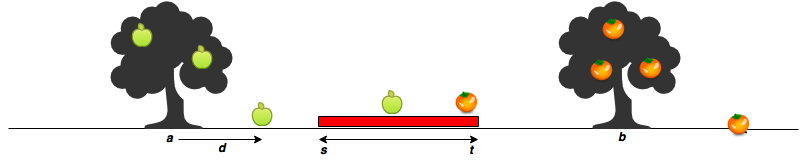# Apple and Orange, Algorithm

Sam’s house has an apple tree and an orange tree that yield an abundance of fruit. In the diagram below, the red region denotes his house, where is the start point and is the end point. The apple tree is to the left of his house, and the orange tree is to its right. You can assume the trees are located on a single point, where the apple tree is at point and the orange tree is at point .When a fruit falls from its tree, it lands units of distance from its tree of origin along the -axis. A negative value of means the fruit fell units to the tree’s left, and a positive value of means it falls units to the tree’s right.

Given the value of for apples and oranges, can you determine how many apples and oranges will fall on Sam’s house (i.e., in the inclusive range )? Print the number of apples that fall on Sam’s house as your first line of output, then print the number of oranges that fall on Sam’s house as your second line of output.

Input Format

The first line contains two space-separated integers denoting the respective values of and .
The second line contains two space-separated integers denoting the respective values of and .
The third line contains two space-separated integers denoting the respective values of and .
The fourth line contains space-separated integers denoting the respective distances that each apple falls from point .
The fifth line contains space-separated integers denoting the respective distances that each orange falls from point .

Constraints

Output Format

Print two lines of output:

1. On the first line, print the number of apples that fall on Sam’s house.
2. On the second line, print the number of oranges that fall on Sam’s house.

Sample Input 0

7 11
5 15
3 2
-2 2 1
5 -6


Sample Output 0

1
1


Explanation 0

The first apple falls at position .
The second apple falls at position .
The third apple falls at position .
The first orange falls at position .
The second orange falls at position .
Only one fruit (the second apple) falls within the region between and , so we print as our first line of output.
Only the second orange falls within the region between and , so we print as our second line of output.


<?php

$handle = fopen ("php://stdin","r"); fscanf($handle,"%d %d",$s,$t);
fscanf($handle,"%d %d",$a,$b); fscanf($handle,"%d %d",$m,$n);
$apple_temp = fgets($handle);
$apple = explode(" ",$apple_temp);
array_walk($apple,'intval');$orange_temp = fgets($handle);$orange = explode(" ",$orange_temp); array_walk($orange,'intval');

$res1=0;$res2=0;
for ($i=0;$i < count($apple);$i++) {
$temp =$a + $apple[$i];
if ($s <=$temp && $t >=$temp ) {
$res1 ++; } } for ($i=0; $i < count($orange); $i++) {$temp = $b +$orange[$i]; if ($s <= $temp &&$t >= $temp ) {$res2 ++;
}
}
echo $res1."\n".$res2;
?>